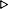ToC DocOverview CGDoc RelNotes Index PermutedIndex
Allegro CLANSI Common Lisp14 Conses14.2 Dictionary of Conses

 14.2.27 revappend, nreconc Function

Syntax:
revappend list tailresult-list

nreconc list tailresult-list

Arguments and Values:
list - a proper list.

tail - an object.

result-list - an object.

Description:
revappend constructs a copy2 of list, but with the elements in reverse order. It then appends (as if by nconc) the tail to that reversed list and returns the result.

nreconc reverses the order of elements in list (as if by nreverse). It then appends (as if by nconc) the tail to that reversed list and returns the result.

The resulting list shares list structure with tail.

Examples:
``` (let ((list-1 (list 1 2 3))
(list-2 (list 'a 'b 'c)))
(print (revappend list-1 list-2))
(print (equal list-1 '(1 2 3)))
(print (equal list-2 '(a b c))))(3 2 1 A B C)TTT

(revappend '(1 2 3) '())(3 2 1)
(revappend '(1 2 3) '(a . b))(3 2 1 A . B)
(revappend '() '(a b c))(A B C)
(revappend '(1 2 3) 'a)(3 2 1 . A)
(revappend '() 'a)A   ;degenerate case

(let ((list-1 '(1 2 3))
(list-2 '(a b c)))
(print (nreconc list-1 list-2))
(print (equal list-1 '(1 2 3)))
(print (equal list-2 '(a b c))))(3 2 1 A B C)NILTT

```

Side Effects:
revappend does not modify either of its arguments. nreconc is permitted to modify list but not tail.

Although it might be implemented differently, nreconc is constrained to have side-effect behavior equivalent to:

``` (nconc (nreverse list) tail)
```

See Also:
reverse, nreverse, nconc

Notes:
The following functional equivalences are true, although good implementations will typically use a faster algorithm for achieving the same effect:

``` (revappend list tail) ==(nconc (reverse list) tail)
(nreconc list tail) ==(nconc (nreverse list) tail)
```

Allegro CL Implementation Details:
None.© Franz Inc. 1998-2019 - File last updated 02-07-2019